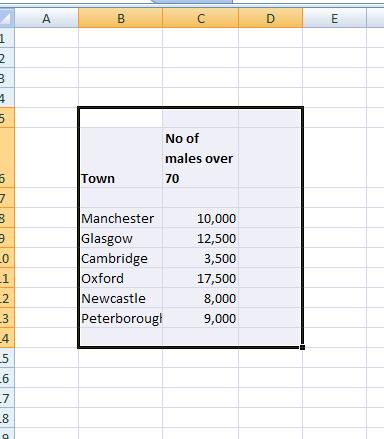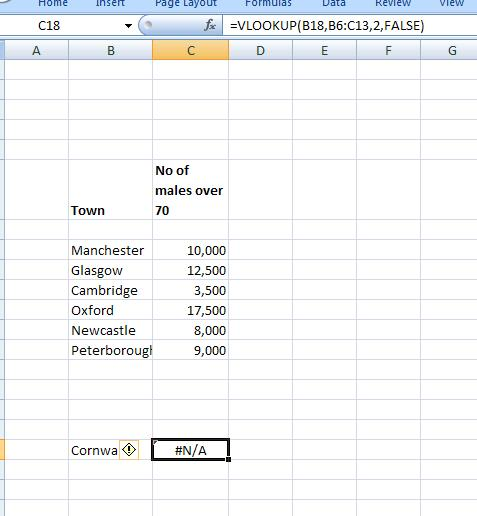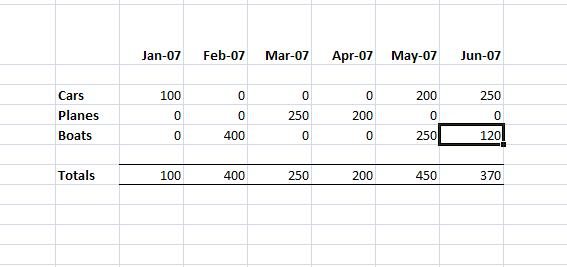# Dealing with NA() Errors

Imagine that we have a table of data such as:And then we do a VLOOKUP on a city that isn’t there we get an N/A error:This is not much of an issue if we have only a single N/A error. However, if we have several N/A (each arising from a separate VLOOKUP) in a table then it can look rather unsightly. In addition any totals that involve these cells also result in the value N/A. In short the N/A error can propagate through the entire workbook:To get around this, Excel has the function ISNA which allows us to check whether or a condition or a cell has the value N/A. It is usually used as part of an IF statement:

B1 =IF (ISNA(A1), 0,A1)

i.e if the cell A1 has the value ISNA then the cell B1 takes the value 0 otherwise it has the value in A1.

Or if we have a VLOOKUP formulae, we can check whether or not it evaluates to NA using the same approach. If does then we take the value 0 otherwise we take the value returned by the VLOOKUP value:

=IF(ISNA(VLOOKUP(B18,B6:C13,2,FALSE)),0, VLOOKUP(B18,B6:C13,2,FALSE))

And if this approach is adopted in the individual rows in the above table we have:Which is much more presentable and the totals now evaluate sensibly.